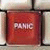## Access 2003, calculating datesTags:
calculating dates
Microsoft Access 2003
I have a database and I need the following to calculate based off a date field. My problem is that regardless if the date entered in that field is the beginning, middle, or end of month, I need another field that will produce a due date, which has to be the end of that month, which could be 6 month, plus some days. See example below. DateReceived = existing field 5/2/08 DueRO = calculated field 10/2/08 (five months from DateReceived) ReportDueFFC = calculated field 11/30/08 (must equal 6 months, plus whatever amount of days necessary to generate the end of month) DaysLate = calculated field (equal number of days past due, using the ReportDueFFC field) I have no problem calculating the number of days, only the ReportDueFCC field. I am not sure how I can generate a date, that vary from DueRO, because that field depends on the DateReceived field. If you have any suggestion, I would greatly appreciate it. Thanks in Advance.

Thanks. We'll let you know when a new response is added.

These are the formulas where B1 is the DateReceived field and the rest are one row below.:

DueRO: =DATE(YEAR(B1),MONTH(B1)+5,DAY(B1))
ReportDueFFC: =DATE(YEAR(B2),2+MONTH(B2),1)-1
DaysLate: =B3-NOW()
———-
DaysLate: =DateDiff(“d”;[ReportDueFFC];Date())

## Discuss This Question: 4 Replies

••